# Basic use of plot_grid()

The plot_grid() function provides a simple interface for arranging plots into a grid and adding labels to them:

require(cowplot)
theme_set(theme_cowplot(font_size=12)) # reduce default font size
plot.mpg <- ggplot(mpg, aes(x = cty, y = hwy, colour = factor(cyl))) +
geom_point(size=2.5)
plot.diamonds <- ggplot(diamonds, aes(clarity, fill = cut)) + geom_bar() +
theme(axis.text.x = element_text(angle=70, vjust=0.5))
plot_grid(plot.mpg, plot.diamonds, labels = c('A', 'B'))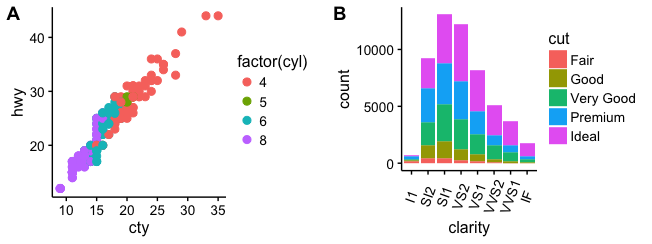If you specify the labels as labels="AUTO" or labels="auto" then labels will be auto-generated in upper or lower case, respectively:

plot_grid(plot.mpg, plot.diamonds, labels = "AUTO")plot_grid(plot.mpg, plot.diamonds, labels = "auto")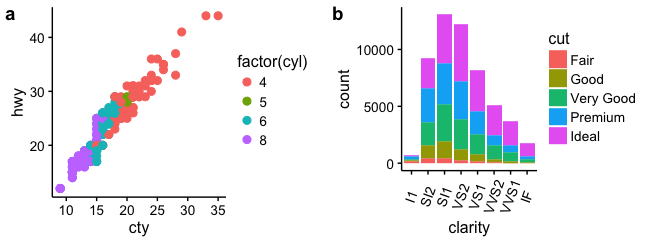By default, the plots are not aligned, but in many cases they can be aligned via the align option:

plot_grid(plot.mpg, plot.diamonds, labels = "AUTO", align = 'h')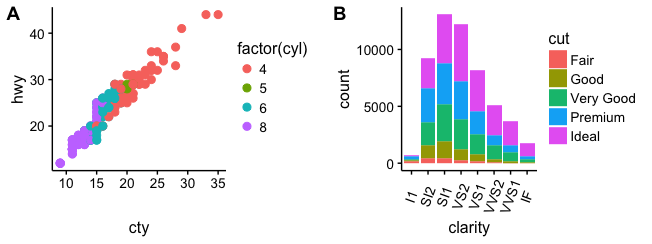plot_grid(plot.mpg, plot.diamonds, labels = "AUTO", ncol = 1, align = 'v')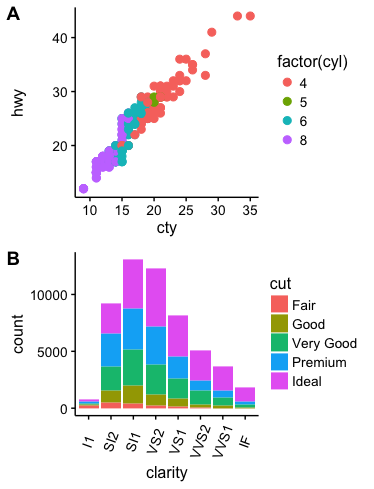In general, only plots that have the same number of visual elements can be aligned. Thus, a faceted and a non-faceted plot cannot be aligned. plot_grid() will know when it can and cannot align plots and will provide a warning in cases alignment is not possible:

plot.iris <- ggplot(iris, aes(Sepal.Length, Sepal.Width)) +
geom_point() + facet_grid(. ~ Species) + stat_smooth(method = "lm") +
background_grid(major = 'y', minor = "none") + # add thin horizontal lines
panel_border() # and a border around each panel
plot_grid(plot.iris, plot.mpg, labels = "AUTO", ncol = 1,
align = 'v') # aligning does not work here
## Warning in align_plots(plotlist = plots, align = align): Graphs cannot be
## vertically aligned. Placing graphs unaligned.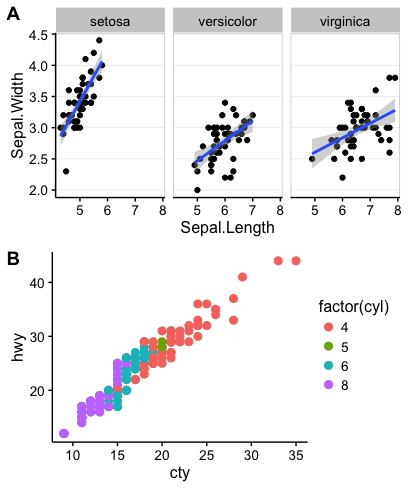In cases where plot_grid() cannot automatcially align plots, you can still align them manually if you have some knowledge of gtable, the internal layouting mechanism used by ggplot2. You simply have to convert both graphs into gtable objects and then adjust the relevant widths or heights. For the plot from the previous example, for example, you could do the following:

require(grid) # for unit.pmax(), unit.list()
g.iris <- ggplotGrob(plot.iris) # convert to gtable
g.mpg <- ggplotGrob(plot.mpg) # convert to gtable

if(getRversion() < "3.3.0"){
# We need to convert the widths to unit lists for the subsequent
# manipulations to be possible.
# Once R 3.3.0 is released, this will not be necessary anymore.
g.iris$widths <- grid:::unit.list(g.iris$widths)
g.mpg$widths <- grid:::unit.list(g.mpg$widths)
}

iris.widths <- g.iris$widths[1:3] # extract the first three widths, # corresponding to left margin, y lab, and y axis mpg.widths <- g.mpg$widths[1:3] # same for mpg plot
max.widths <- unit.pmax(iris.widths, mpg.widths) # calculate maximum widths
g.iris$widths[1:3] <- max.widths # assign max widths to iris gtable g.mpg$widths[1:3] <- max.widths # assign max widths to mpg gtable

# plot_grid() can work directly with gtables, so this works
plot_grid(g.iris, g.mpg, labels = "AUTO", ncol = 1)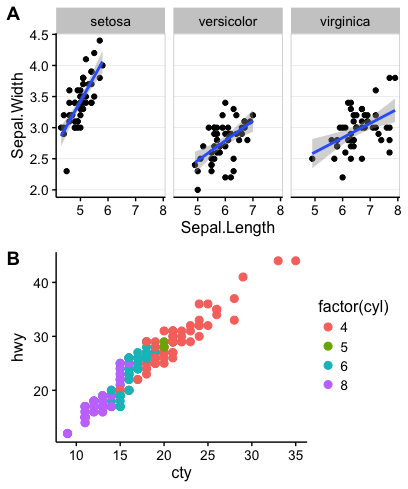Notice how now the y axes in plots A and B are located at exactly the same horizontal position.

# Fine-tuning the plot appearance

You can adjust the label size via the label_size option. Default is 14, so larger values will make the labels larger and smaller values will make them smaller:

plot_grid(plot.mpg, plot.diamonds, labels = "AUTO", align = 'h', label_size = 12)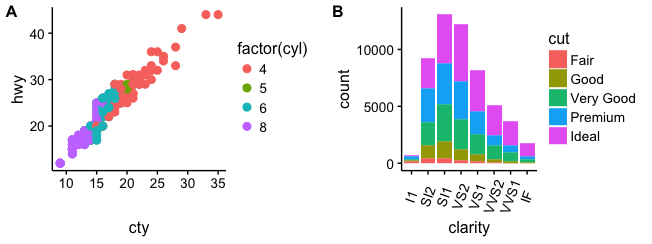Labels can be moved via the hjust and vjust options. More negative numbers move the labels to the right or up, respectively:

plot_grid(plot.mpg, plot.diamonds, labels = "AUTO", align = 'h', label_size = 12,
hjust = -1, vjust = 2.5 )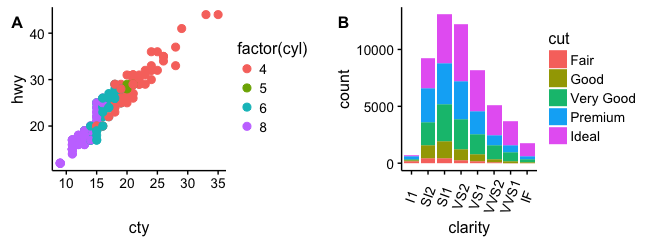It is possible to adjust individual labels one by one by passing vectors of adjustment values to the options hjust and vjust.

You can also adjust the relative widths and heights of rows and columns:

plot_grid(plot.mpg, plot.diamonds, labels = "AUTO", align = 'h', rel_widths = c(1, 1.3))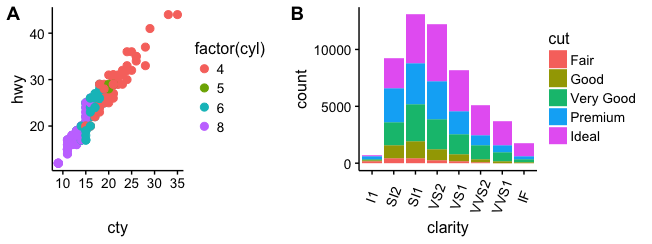Finally, it is possible to scale the overall size of the plots up or down. This can be useful if the plots have too much or too little margin. Note that scale values >1 will not work if they move any part of a plot outside the entire plot area.

plot_grid(plot.mpg, plot.diamonds, labels = "AUTO", align = 'h', rel_widths = c(1, 1.3), scale = 0.95)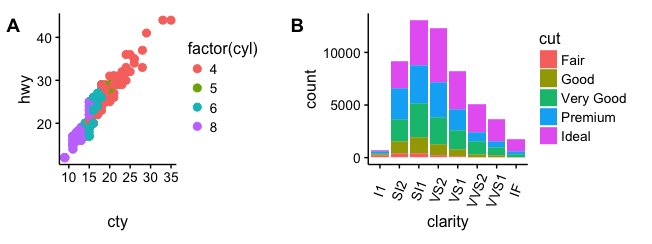# Nested plot grids

If you want to generate a plot arrangement that is not a simple grid, you may insert one plot_grid() plot into another:

bottom_row <- plot_grid(plot.mpg, plot.diamonds, labels = c('B', 'C'), align = 'h', rel_widths = c(1, 1.3))
plot_grid(plot.iris, bottom_row, labels = c('A', ''), ncol = 1, rel_heights = c(1, 1.2))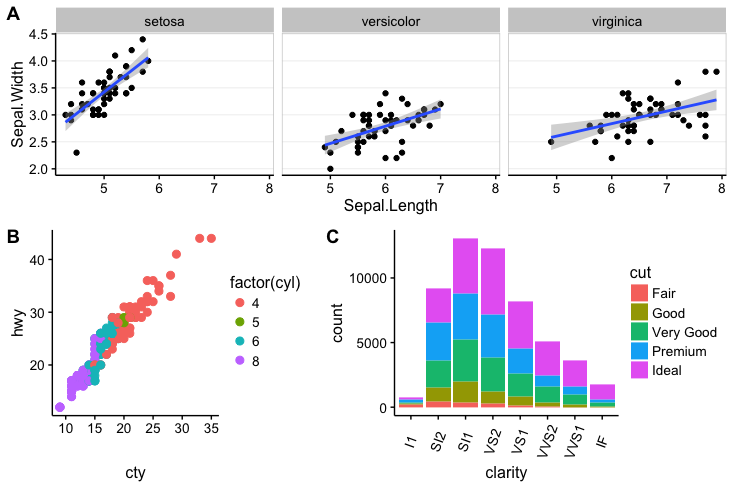(Notice how we used rel_heights to make to bottom row higher than the top row, and scale to introduce a little extra white-space around the plots. Also, we can’t auto-generate the labels in this case.) Alignment is a bit tricky in this case, though, and we won’t discuss this here. In principle, it can again be achieved by appropriately manipulating the underlying gtables.